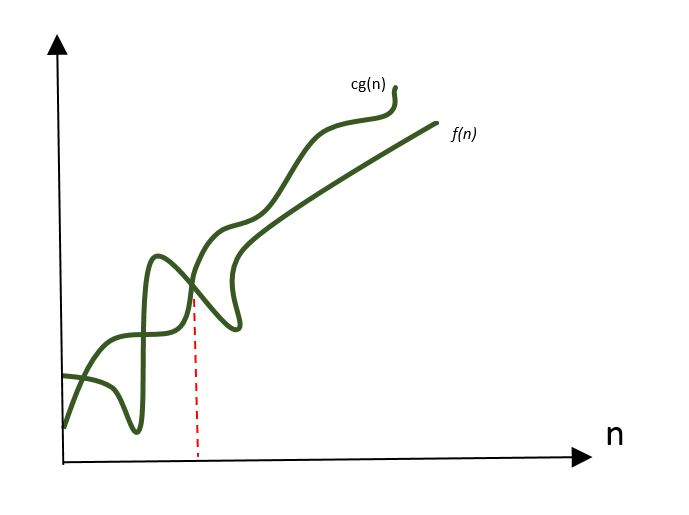Open in App
Not now

# GATE | GATE-CS-2000 | Question 40

• Last Updated : 28 Jun, 2021

Consider the following functionsWhich of the following is true?
(A) h(n) is O(f(n))

(B) h(n) is O(g(n))
(C) g(n) is not O(f(n))
(D) f(n) is O(g(n))

Explanation: Big-oh notation:

Let f and g be two functions defined on real number. One writes f(n) = O(g(n)) if there is a positive constant M such that for all sufficiently large values of n, the absolute value of f(n) is at most M multiplied by the absolute value of g(n). That is, f(n) = O(g(n)) if and only if there exists a positive real number M and a real number n0 such that f(n)≤M(g(n)), for all n≥n0.In Big-oh notation, we only make comparison between two functions by considering larger values of n. To solve question like this we can take larger value of n and then compare the values of different function.

f(n) = 3(n^32)=3*(2^10)^32=3*2^320

g(n) = 2^320

h(n)=1024!

So relation between the functions can be:

1.f(n) and g(n) are of same order, so f(n) is O(g(n)) and g(n)=O(f(n)). Hence option C is wrong.
2.h(n) is n! Which is of higher order than f(n) and g(n). So options A and B are wrong.

This solution is contributed by Nirmal Bharadwaj

Quiz of this Question

My Personal Notes arrow_drop_up
Related Articles